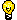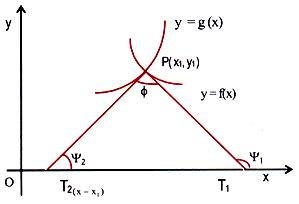Click to Chat

1800-1023-196

+91-120-4616500

CART 0

• 0

MY CART (5)

Use Coupon: CART20 and get 20% off on all online Study Material

ITEM
DETAILS
MRP
DISCOUNT
FINAL PRICE
Total Price: Rs.

There are no items in this cart.
Continue Shopping```Angle Between Two Curves

Angle Between Two CurvesLet there be two curves y = f1(x) and y = f2(x) which intersect each other at point (x1, y1). If we draw tangents to these curves at the intersecting point, the angle between these tangents, is called the angle between two curves.

Let m1 = (df1 (x))/dx |(x=x1) and m2 = (df2 (x))/dx |(x=x1)

And both m1 and m2 are finite.

The acute angle between the curves is given by

θ = tan-1 |(m1-m2)/(1+m1 m2 )|Note:  (p - q) is also an angle between lines.

If m1 (or m2) is infinity the angle is given by θ = |π/2-θ1 | where

θ1 = tan-1 m2 (or tan-1 m1)

In the figure given below, f is the angle between the two curves,which is given by

f = Ψ1 - Ψ2

=> tanΦ = tan (Ψ1 - Ψ2)

= (tan Ψ1-tan Ψ2)/(1+tan Ψ1 tan Ψ2 ),

where tan Ψ1 = f'(x1) and tan Ψ2 = g'(x1).Two curves are said to cut each other orthogonally if the angle between them is a right angle, that is, if f = 90o, in which case we will have,

tanΨ1 tanΨ2 = -1.

Two curves touch each other if the angle between the tangents to the curves at the point of intersection is 0o, in which case we will have,

tanΨ1 = tanΨ2.

Illustration:

Show that the curves ax2 + by2 = 1 and cx2 + dy2 = 1 cut each other orthogonally if, 1/a-1/b=1/c-1/d.

Solution:

Let the two curves cut each other at the point (x1, y1). Then

ax12 + by12 = 1                                                           ...... (1)

and cx12 + dy12 = 1.                                                     ...... (2)

From (1) and (2), we get

(a - c)x12 + (b - d)y12 = 0.                                           ...... (3)

Slope of the tangent to the curve ax2 + by2 = 1, at (x1, y1) is given by

tan Ψ1 = [dy/dx](x1,y1) = -ax1/by1 .

Slope of the tangent to the curve cx2 + dy2 = 1 at (x1, y1) is given by

tan Ψ2 = [dy/dx](x1,y1) = -cx1/dy1. If the two curves cut orthogonally, we must have,

(-ax1/by1 )(-cx1/dy1 ) = -1 => acx12 + bdy12 = 0.                          ...... (4)

From (3) and (4) we have

(a-c)/ac=(b-d)/bd => 1/a-1/c=1/c-1/d.

Illustration:

Prove that the tangent lines to the curve y2 = 4ax at points where x = a are at right angles to each other.

Solution:

y2 = 4ax

When x = a

y2 = 4a2

=>   y = +2a

points are (a, 2a) and (a, - 2a)

Slope of the tangent of the curve y2 = 4ax is

y dy/dx = 4a

dy/dx = 2a/y

for y = 2a, dy/dx = 1 = m1

for y = -2a dy/dx = - 1 = m2

m1m2 = -1

To read more, Buy study materials of Applications of Derivatives comprising study notes, revision notes, video lectures, previous year solved questions etc. Also browse for more study materials on Mathematics here.
```### Course Features

• 731 Video Lectures
• Revision Notes
• Previous Year Papers
• Mind Map
• Study Planner
• NCERT Solutions
• Discussion Forum
• Test paper with Video Solution